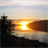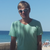# New to Qlik Sense

If you’re new to Qlik Sense, start with this Discussion Board and get up-to-speed quickly.

Announcements
Skip the ticket, Chat with Qlik Support instead for instant assistance.
cancel
Showing results for
Did you mean:Contributor II

## Sum total absolute value

Hi everyone,
I have a problem when I calculate sum total of my data. I want to take fabs for each row before sum total

Like my example: x=-1,2,-3,4,-5 (each element is value of each day )
I want sum total= fabs(-1)+fabs(2) + ...+ fabs(-5)
My expectation = 15

4 RepliesMaster II

Where do you want to show this value? In a textbox?

```Load *, Fabs(X) as Fabs;
Date,  X
01/01/2018, -1
02/01/2018, 2
03/01/2018, -3
04/01/2018, 4
05/01/2018, -5
];```

And in Textobject use this to get TOTAL of 15:

`sum(TOTAL Fabs)orsum(TOTAL Fabs(X))`Contributor II
Author
Thanks for your help, actually i use it for textbox. But do you have other way to solve by expression , not using load data ?Contributor II
You can you use the following expression:
=SUM(FABS(x))